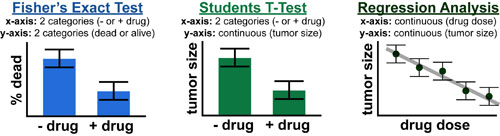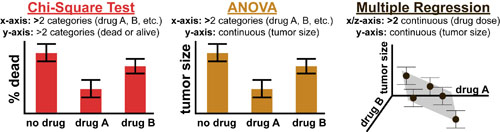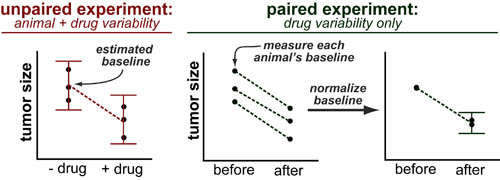# Choosing a Statistical TestThe number and type of possible statistical tests in experimental science can be bewildering. Luckily choosing the right test can be made alot easier by first considering the question: Is your data categorical or continuous? Categorical data is typically percentage or frequency data binned into 2 or more categories or names. Continuous data is typically measurement data where there is a well defined relationship between the values (i.e. numerical data)1,2

To choose the right type of statistical test you must consider what type of data you have on your x-axis (independent variable/test conditions/etc.) and y-axis (dependent variable/measurement conditions/etc.). The figure above presents data from the same hypothetical experiment (of the efficacy of an anti-cancer drug) and shows how the choice of statistical test depends on the method of data presentation.When dealing with more than two conditions/categories/independent variables the figure above outlines the appropriate tests statistics (depending on the method of data presentation).

While “continuous” vs “categorical” data convers the major categories of statistical tests there are more specialized tests that are additional sub-types or specially developed for specific experiments:

##### Sub-types of above tests:
• one-tailed vs two tailed: do you expect a directional effect? In “two-tailed” tests, a difference in either direction (from the “null”/”baseline”/”negative control”) can satisfy the “statistical significance” criterion. If, however, you know the direction of your expected change (for example: more drug should equal less tumor) you can improve the sensitivity of your test by using a one-tailed test.
• paired vs unpaired tests: before/after or randomized test? “Unpaired tests” are used for experiments where “+ drug animals” and “- drug animals” are randomly selected. “Paired” look at “+ and – drug” within the same test animal. This reduces animal-to-animal variability and results in a much more sensitive test.##### Specialized Test-types
• Ranked Tests: Ranked tests are used for data that is in between the “continuous” and “catagorical” types. In ranked tests, the order of the measurements is known (1st, 2nd, 3rd, etc.) but exact values are not known (e.g. what is the difference between 1st and 2nd vs 2nd and 3rd?). Ranked tests are not very common but can be very powerful when properly used. Examples of ranked tests include: Wilcoxon’s rank sum test, Kruskall-Wallis test.
• log-rank test: special type of ranked test which examines differences in animal survival time

REFERENCES:1.Rupali Gund